# Intrinsic attenuation from inhomogeneous waves in a dissipative anisotropic poroelastic medium

## Abstract

A procedure is suggested to translate the quality factor of a plane harmonic attenuating wave in a general anisotropic elastic medium into its phase velocity and two finite non-dimensional attenuation parameters. A chosen value of the quality factor of an attenuated wave in the dissipative medium is used to specify its complex slowness vector for a general direction of propagation. In this specification, one of the attenuation parameter identifies the component of intrinsic attenuation along the direction of propagation of wave, i.e., homogeneous propagation of wave. Another parameter represents the component of attenuation in the direction orthogonal to propagation direction. It measures the deviation from homogeneous propagation and is termed as the inhomogeneity strength of the attenuated wave. These attenuation parameters alongwith phase velocity are used to calculate the rate of decay of amplitude of the attenuated wave along any given direction in propagationattenuation plane. Biot’s theory is used to study the propagation of four attenuating waves in an anisotropic poroviscoelastic medium in the presence of initial stress. For each wave, the specification of complex slowness vector is obtained in terms of its phase velocity and two attenuation parameters. Numerical results show that the attenuation contribution from the homogeneous propagation (of any of the three faster waves) is only a little in the total attenuation of any of these attenuated waves. The effects of the changes in anisotropy-type, initial-stress, frequency, fluid-viscosity, viscous characteristic length, and anelasticity of porous frame on the attenuation are also studied. It is found that though they affect the phenomenon of wave propagation and wave characteristics, major contribution to total intrinsic attenuation comes from the inhomogeneous propagation of the attenuated wave.

## 1. Introduction

The accurate analysis of observed seismic attenuation is important for the advancement in knowing the structure of the earth. This is particularly true in exploration industry and in the investigation of tectonic stress and failure where small scale fracturing and flow of fluid into the fractures is important. The confined stresses and shale anisotropy helps in predicting flow path for improved oil recovery and designing hydraulic fracturing schemes. The flow mechanism to equilibrate fluid pressure produces a great deal of seismic attenuation at high frequency. The total attenuation inferred from the seismograms is the sum of intrinsic attenuation and scattering attenuation. Major contribution comes from the intrinsic attenuation (Sams et al., 1997), which represents the internal frictions between the adjacent material grains not elastically bonded. The seismic data from sedimentary regions exhibit more intrinsic attenuation that can be explained through existing theoretical models (Pride et al., 2004). Analysis of seismic data suggests that hydrocarbon deposits are often associated with higher than usual values of attenuation, but this is generally ignored during amplitude-versus-offset (AVO) analysis. These larger attenuations are, generally, translated into the stronger sources of attenuation, i.e., intrinsic physical processes, such as the interactions between the solid and the fluid, grain friction etc. Chapman et al. (2006) studied the reflections from the interface between the medium based on squirt flow concept and an elastic overburden. They found that reflection coefficient varies with frequency and the impact of this variation depends on the AVO behaviour at the interface. Liu et al. (2007) approved the use of difference in velocity and attenuation anisotropy to understand the mechanisms and to extract the additional information about subsurface fracture systems.

Whatever be the sources of attenuation of seismic waves in sedimentary rocks, the mathematical models are often required to explain the (rate of) decay of amplitude of waves propagating away from the source. This demands a much deeper insight into the process of wave propagation in realistic models of sedimentary rocks in the crust. These rocks can, more closely, be modeled as fluid-saturated porous solids pervaded by aligned cracks. The fluid-saturated microcracks are highly compliant and crustal rocks respond immediately to the small changes in differential stress (i.e., difference between confined tectonic stress and fluid pressure). Hence, a pre-stressed anisotropic porous solid makes a much realistic geophysical model to be used for seismic characterization of sedimentary or reservoir rocks. In particular, such a composite physical model facilitates the parametric studies of the influence of various measurable physical properties of the medium (i.e., porosity, permeability, pore-fluid viscosity, frame anelasticity, initial-stress, elastic/hydraulic anisotropy, pore-size, etc.).

Biot (1956) used Lagrange’s equations to derive a set of coupled differential equations that governs the propagation of motions of solid and fluid particles in a fluidsaturated porous medium. Biot (1962a, b) extended the acoustic propagation theory in the wider context of the mechanics of porous media and developed the new and simplified derivations of the fundamental equations of poroelastic propagation. A little later, Biot (1963) introduced the effect of initial stress in the mechanics of porous media and developed the basic equations. Stoll and Bryan (1970) used Biot’s theory (1962a, b) to study the wave attenuation in saturated sediments. In the sedimentary rocks, the anelastic nature alone may not be able to explain the observed attenuation. The confining stresses and wave-induced flow of viscous-fluid in pores and cracks are another important factors. In an initially-stressed porous medium of transverse isotropy, Sharma and Gogna (1991, 1993) studied the propagation of SH waves. Sinha et al. (1995) considered inhomogeneous pre-stresses in the porous medium resulting from the pressurized fluid in borehole. Sams et al. (1997) measured the large intrinsic attenuation in the stratified sequence of water-saturated porous sediments. Attenuation anisotropy in reservoir intervals is generally stronger than in overburdens. Numerical examples studied by Sharma (2005a) suggest that effect of initial-stress may be much more on attenuation as compared to velocities. Shapiro and Kaselow (2005) developed a formalism that describes the elastic moduli, anisotropy and porosity of rocks as functions of confining stress and pore pressure.

An earlier study of author (Sharma, 2005b) shows that, compared to velocity, the attenuation is more sensitive to the inhomogeneity of waves propagating in dissipative anisotropic poroelastic medium. Much earlier, Winterstein (1987) related the quality factor (Q) variations in a multilayered medium to the inhomogeneity (angle between propagation direction and direction of maximum attenuation) of the attenuating waves. In another study, Carcione (1999) suggested that the differences in amplitude variation with offset (AVO) of waves transmitted at ocean bottom depend not only on the properties of the medium but also on the inhomogeneity of the wave. This implies that the propagation of inhomogeneous waves may be able to explain, mathematically, the larger attenuation of seismic waves in sedimentary regions. Importance of inhomogeneous waves in single-phase viscoelastic media are found in Borcherdt (1977, 1982) and Cerveny and Psencik (2005a, b). Experimental results (Borcherdt et al., 1986; Hosten et al., 1987) confirm the generation and existence of inhomogeneous body waves and the differences in their physical characteristics from elastic body waves. Carcione (2006) studied the Rayleigh-window effect to explain the role of inhomogeneous waves in amplitude reduction of the reflection coefficient of the ocean bottom. The theory about inhomogeneous body waves in porous media has been given in Carcione (2007).

A mathematical model of wave propagation should explain the attenuation through the decay of amplitude away from source, i.e., AVO. The amplitude decay of a plane harmonic wave in dissipative media is derived from the specification of its complex slowness vector. A general analysis of attenuation requires the complex slowness vector to represent the propagation of inhomogeneous waves. The work presented proposes a procedure to relate the quality factor of attenuation to the inhomogeneity of the attenuated wave in a dissipative medium. The specification of complex slowness vector of the attenuated elastic wave provides its phase velocity, homogeneous attenuation and inhomogeneity strength, which can be used to calculate the decayrate of amplitudes of displacements of material particles. The procedure proposed is used to study the propagation of inhomogeneous waves in an anisotropic, initially-stressed, anelastic porous solid medium saturated with viscous fluid. The numerical examples considered certifies the direct relationship between quality factor of attenuation and inhomogeneity strength of an attenuated wave.

## 2. Inhomogeneous PlaneWaves

The general plane waves propagating in a dissipative medium are inhomogeneous waves (Borcherdt, 1982). These waves exhibit physical properties those are different from the body waves in elastic media. According to Caviglia et al. (1990), a plane wave is said to be inhomogeneous if its slowness vector is complex valued. The real and imaginary parts of complex slowness vector are termed as propagation vector and attenuation vector, respectively. In general, the inhomogeneity of an attenuating wave is represented through the difference in the directions of its propagation vector and attenuation vector. In other words, an angle between equi-amplitude plane and equi-phase plane of an attenuating plane wave represent its inhomogeneous character.

Complex slowness vector of an attenuating wave is expressed as(1)

where, propagation direction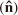is normal to equi-phase plane and direction of maximum attenuation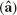is normal to equi-amplitude plane. The angle (γ) betweenanddefines the inhomogeneous character of the attenuating wave with (κ) being its attenuation-propagation ratio. The real quantity υ is the phase velocity of wave propagating along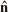and suffering maximum attenuation along. In the following procedure we are using a non-dimensional finite parameter (δ) to define an inhomogeneous wave. It measures the deviation of an inhomogeneous wave from its homogeneous version (represented by, δ = 0) and is termed as inhomogeneity strength. To assign a role to δ in propagation phenomenon, consider a plane formed by two orthogonal unit vectorsandsuch that it contains the attenuation vector. Then, the expression (1) of complex slowness vector (p) changes to(2)

where, β is the component of attenuation vectoralong the direction of propagation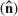. Hence, β identifies the homogeneous attenuation of an attenuated wave. The another parameter δ, which is the component of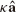along orthogonal direction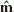, identifies the inhomogeneous attenuation. In fact, in the maximum intrinsic attenuation of an attenuated wave, the contributions of homogeneous propagation (i.e. γ = δ = 0) and evanescent propagation (i.e. γ = π/2) are defined by the ratios β/υ and δ/υ, respectively. So, the phase velocity (υ) and attenuation parameters β, δ) are the (real) unknowns, to be determined for given direction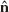and orthogonal direction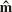in a dissipative medium. Using a substitution p = N/V, such that N.N = 1, of complex slowness vector in the propagation of harmonic plane waves in any anisotropic (dissipative) medium provides a system of Christoffel equations. This system can be solved into an algebraic equation, which provides the values of complex velocity V for any given N. Using this relation among p, V and N, Eq. (2) yields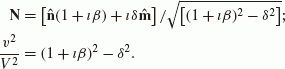(3)

Denote the complex quantity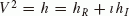so that we have a complex equation(4)

which, on resolving, yield two real equations as follows.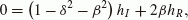(5)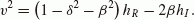(6)

Combining these two equations implies that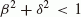ensures a positive value for υ2 when hR is positive. Defining the quality factor (Q) of attenuation as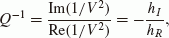(7)

Eq. (5) yields(8)

This last relation implies that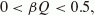(9)

which restricts the value of β for any attenuating wave with a given value of Q. This implies that even for a highly attenuating inhomogeneous wave (i.e., Q-1 ≤ 0.1) the contribution of its homogeneous propagation to the total attenuation (κ < 1) may be less than five percent. However, for the restricted homogeneous propagation, i.e. δ = 0, the whole attenuation is represented only with β. But, in this case Q-1 is found to be very small (Sharma, 2005a) and then, from Eq. (8), β (< 0.5Q-1 will also be very small. Moreover, in viscoelastic solids, it is observed that for δ = 0, the value of β is found to be very near to zero (Sharma, 2008a). Similar results are obtained in the numerical example of the present study.

It is calculated that Eq. (5) has a root in the range of β given by (9) or an equivalent range of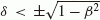in (-1, 1). To calculate attenuation parameters (β, δ) and phase velocity (υ), the various relations derived above are written in terms of Q as follows.(10)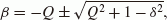(11)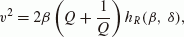(12)(13)

For a chosen value of Q, Eq. (11) provides β as a function of δ. The sign ± is chosen to ensure the same sign for β and Q as required in (9). Then the left side of Eq. (10) becomes a non-algebraic function of only one unknown, i.e. δ. A numerical method such as bisection method or Newton-Raphson method can find δ as its root in (-1, 1). Thus obtained δ and the chosen value of Q are used in relations (11)–(13) to calculate the values of β, υ and γ. A possibility may not be ruled out that for an arbitrary value of Q or even for a genuine looking value of Q, the value of hR may not be positive for some direction of propagation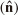. Then, Eq. (10) may not provide a valid solution for δ.

In general, it is expected that for a chosen direction of propagationof an attenuated wave, an intuitively chosen value of quality factor Q yields the valid values for υ, β and δ. These values together are sufficient to calculate the variations of amplitudes (of displacements) of material particles with offset. It is calculated that along any given direction inplane making an angle (say) α with, an amplitude reduces to half at every distance r, given by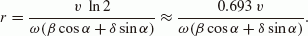(14)

Note that for any attenuating wave in the dissipative medium, the distance r is inversely proportional to its frequency and directly proportional to its phase velocity. The relation (14) indicates that there may exist a direction, making an anglewith, along which the distance r will be infinite. This means the harmonic disturbance propagating as an inhomogeneous plane waves may not decay along this direction.

Finally, we have a system of equations to calculate the unknowns γ, δ, υ and inhomogeneity angle γ from a given value of Q for an attenuating wave in a dissipative medium. In other words, the procedure explained above is capable of translating the attenuation quality factor for a wave in any dissipative anisotropic elastic medium into its propagation and attenuation characteristics as inhomogeneous wave. However, this procedure requires an algebraic system for the medium so as to calculate the complex function h (= V2) corresponding to a given complex vector N. In the next section, such a system is derived for a general and realistic dissipative (pre-stressed anisotropic poroelastic) medium.

## 3. Pre-Stressed Anisotropic Porous Solid

### 3.1 Fundamental equations

Following Biot (1962a), a set of differential equations governs the particle motion in a general anisotropic porous solid frame, of anisotropic permeability, saturated by a viscous fluid. Consider this medium to be under homogeneous initial stress defined through Sij the components of a symmetric tensor. The absence of body forces in the porous medium ensures that fluid density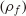and initial fluid pressure will be uniform. Following Biot (1963), the constitutive equations governing the dynamics of porous media under initial stress, in the absence of body forces, are given by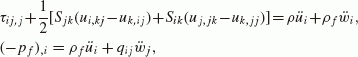(15)

where. The components Ui and ui define the displacements of particles of pore-fluid and solid frame, respectively. f is the porosity of the solid matrix. Indices can take the values 1, 2 and 3. Summation convention is valid for repeated indices. The comma (, ) before an index represents partial space differentiation and dot over a variable represents partial time derivative. The ρ and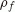are the densities of porous aggregate and pore-fluid, respectively. The symmetric tensor q, controls the inertial as well as viscous coupling between fluid and solid phases. The effect of viscosity (μ) of pore fluid is restricted only to dissipation due to the relative motion between fluid and solid particles. This implies that the attenuation comes from the wave-induced flow of viscous fluid in the pores. Following Johnson et al. (1987) and Albert (1993), q can be written in terms of anisotropic permeability (K) of solid matrix, and tortuosity of poresfor non-viscous fluid, as(16)

where, I is an identity matrix of order three and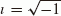. Writing the permeability tensor K(=koKa) with maximum permeability value ko, the dissipation matrix d is defined as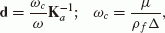(17)

where,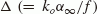is a parameter with dimension of permeability. ω is the angular frequency of plane harmonic wave with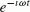time dependence. The expression of d, in Eq. (17), is valid for low frequency, when the flow in pores is of Poiseuille type. For high frequency, the viscosity μ is multiplied by a frequency dependent correction factor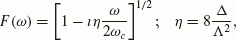(18)

where, the viscous characteristic length ι represents the configuration of pores. The value of non-dimensional parameter η is around 1, for most of the porous media (Albert, 1993). Hence, the dissipation d in the medium becomes an explicit function of two parameters ω/ωc and η. In expression (17) of dissipation matrix d, the dynamic tortuosity of pores is expressed as an analytic function of frequency. This explicit frequency dependence of tortuosity was, however, constructed from the results for the low- and high-frequency behaviours. Hence, the analytic function represented the correct behaviour only at these frequency extremes and an error of upto 10% was observed for intermediate frequencies (Johnson et al., 1987).

The incremental stress components τij and fluid pressure(Biot, 1962a) are expressed as(19)

where, the fourth-order tensor cijkl represents the elastic properties of the porous aggregate. The symmetric tensor of elastic parameters mij represents the elastic coupling between fluid and solid phases of the porous aggregate. Elastic constant R measures the pressure exerted on fluid to saturate the porous solid frame. In an initially-stressed medium, the existence of energy density function requires (Biot, 1939) the elastic tensor cijkl to satisfy a relation, given by(20)

where, δij is Kronecker delta.

### 3.2 Harmonic plane waves

For the propagation of plane harmonic waves, write the displacement components as(21)

where, (p1, p2, p3) is slowness vector p. In terms of phase velocity V, the slowness p = N/V, such that complex (or dual) vector N = (N1, N2, N3) satisfies N.N = 1. The harmonic solution (21), through relations (19), is used in equations of motion (15) to obtain (Sharma, 2005a) a system of six linear homogeneous equations in Ej, Fj; (j = 1, 2, 3). This system is manipulated algebraically into two sub-systems, which represent the dynamics of wave propagation in a fluid-saturated porous solid under initial stress. One of them relates the two forms of displacements, i.e., (w) and (u). The other one defines the modified Christoffel equations for the medium as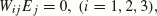(22)

where, matrix W is the same as in Sharma (2005b). The Christoffel equation is just the elastic wave equation transformed over space and time. It specifies the phase velocity and polarization of each plane wave component in frequency domain. For the existence of a non-trivial solution, the modified Christoffel equation is solved (Sharma, 2005b) into a quartic equation in h (= V2), given by(23)

The coefficients Cj in (23) are complex due to the viscosity of pore-fluid and anelasticity of the solid frame in the porous aggregate. The four complex roots (say, hj ; j = 1, 2, 3, 4) of this equation represent the propagation of four attenuating waves in the medium. The complex velocities of the four waves, given bywill be varying with phase vector N. These waves, with velocities represented by Vj, (j = 1, 2, 3, 4), may be called the qP1-, qS1-, qS2-, qP2-waves, respectively. The prefix ‘q’ is used to represent the quasi-longitudinal (qP) or quasi-transverse (qS) characters of the waves in the non-dissipative medium (Sharma, 2008b, 2009). The vector N with real components implies the same directions for propagation and attenuation vectors of these waves and, hence, make them homogeneous waves. However, the propagation of inhomogeneous waves can, only, be explained with N as a complex (dual) vector. Then, the slowness vector p = N/V of each wave is resolved (Section 2) to calculate its phase velocity and attenuation parameters.

## 4. Numerical Examples

The general mathematical model explained in previous sections provides a procedure to specify the complex slowness vectors for the propagation of four inhomogeneous waves in a saturated poroelastic solid under initial stress. The complex slowness vector of each inhomogeneous wave is constituted by the values of phase velocity (υ) and attenuation parameters (β, δ). Numerical examples are studied to analyze the variations of υ, β and δ with propagation directionfor chosen values of Q, η, ω/ωc involved in the mathematical model. The numerical model is considered for North-Sea sandstone, a general anisotropic porous reservoir rock of density ρ = 2216 kg/m3 and porosity(Rasolofosaon and Zinszner, 2002). The interconnected pores of tortuosity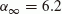and elastic constant R = 5 GPa, are filled with a viscous fluid of density 1000 kg/m3. The elastic constants for this saturated sandstone are defined in Appendix.

For a general direction (θ, φ), in three-dimensional space, the propagation directionis defined by (sin θ cos φ, sin θ sin φ, cos θ). A vertical plane, fixed by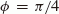, is considered the propagation-attenuation plane for this study. In this plane, the orthogonal vector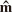is considered alongand θ, varying from 0 to 90°, represents propagation direction. Fixed values are assumed for frequency ω = θc and parameter η = 1.

Figure 1 exhibits the variations of propagationattenuation characteristics (υ, β, δ) for qP1 wave with phase direction (θ). These variations exhibit the anisotropic character of propagation and attenuation of qP1 wave in the medium considered. The three different values for quality factor (Q = 60, 80, 100) provide liberty in selecting an appropriate Q for qP1 wave. It is noted that the phase velocity increases slightly with increase of Q. Both the attenuation parameters (β, δ) increase with the increase of Q. An important observation is that for qP1 wave, the value of β, in general, is not more than five percent of δ. Another important observation may be the sign change of inhomogeneity parameter (δ) for the values of θ in (80°, 90°). The effect of Q as well as θ on υ and β is observed more in this pocket of propagation directions. It is felt that this step in δ must be a discontinuity, i.e., there may be some θ values in this pocket for which the chosen quality factor (Q) cannot be achieved through the valid values of υ, β, δ. Else, there must exist a propagation direction for which δ vanishes, which represents a homogeneous propagation of qP1 wave. But β alone with its restricted value may not be able to justify the attenuation represented by the chosen value of Q. In other words, it is a kind of critical angle which may represent the forbidden direction for propagation of attenuated wave with chosen value of Q in the model considered. For propagation beyond this angle, the inhomogeneity parameter δ with reverse sign yields the genuine value of β, which satisfies the required restriction (9).

In Fig. 2, the phase velocity of qS1 wave increases with the increase of Q value from 20 to 40. For the same increase of Q, the attenuation parameter β decreases but δ increases. The effect of changes in Q is more clear on the characteristics of qS1 wave as compared to qP1 wave in Fig. 1. The behavior of variations for propagation direction θ (80°, 90°) is same as that of qP1 wave. In general, the qS1 wave have a quite larger β than qP1 wave. Hence, the values of δ, even for large Q, may not be more than ten times that of β.

Compared to qS1 wave in Fig. 2, any change in the value of Q in (40, 60) has a very little effect on the phase velocity of qS2 wave (Fig. 3). To any change in Q, the attenuation parameter β is more sensitive than δ. However, for most of the propagation directions, the smaller values of β may not be more than 10 percent of the corresponding values of δ. The pocket of discontinuity in propagation direction for qS2 wave lies for some values of θ (75°, 85°).

The pocket of discontinuity in the propagation direction of qP2 wave is noted (Fig. 4) for θ (20°, 30°). Except in this pocket, an increase in the values of Q (0.95, 1.15), decreases the values of phase velocity (υ) and homogeneous attenuation (β). However, the value of δ increases with an increase in Q. This slowest (qP2) wave is different from other three faster waves in the value of β, which is very much to the order of δ. This wave may be identified as Biot wave and all of its characteristics (υ, β, δ) are quite sensitive to any change in the quality factor (Q).

In the numerical results discussed above it is noticed that, for three faster (qP1, qS1, qS2) waves, the value of β is much smaller than δ. Moreover, this smaller values of β is not the property of the physical parameters chosen for the elastic model used in numerical computation. To ascertain this, the numerical results were calculated for a numerical example with different physical parameters. Dolomite reservoir rock (Rasolofosaon and Zinszner, 2002) of porosity, tortuosity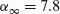, density 2423 kg/m3 and R = 10 GPa was considered as an anisotropic porous solid saturated with water. The elastic tensor and permeability tensor for dolomite are given in Appendix. The variations of β and δ with θ were calculated for all the four waves with different values of Q. The values of β/δ for three faster waves in dolomite were not much different from those observed for sandstone in Figs. 1 to 3.

Now, for three faster waves, we have. It can be calculated from (7) that the quality factor (Q) of attenuation is given by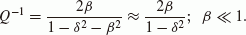(24)

This implies that a large value of Q-1 is obtained when value of δ is very near to 1. This further calculates angle γ to be approaching π/2 and, hence, represents the propagation of nearly evanescent waves. This leads to an interpretation that, in the initially-stressed dissipative anisotropic porous medium, the quality factor (Q-1) of an attenuating wave is a direct diagnostic of its inhomogeneity strength (δ or γ). Similar observation may be found in Carcione (2007) for a dissipative medium even in the absence of initial stress.

To strengthen the claim on relation (24), the role of another parameter (η), which affects the dissipation directly through pore structure, in changing the homogeneous attenuation (β) was analysed. It was found that any change in the value of η around 1 has almost no effect on the values of β and δ for three faster waves. The values of Q chosen for qP1, qS1 and qS2 waves were 80, 30 and 50 respectively. Another factor that may affect the character of an attenuating wave in a dissipative medium is the wave frequency ω. The values of β and δ of three faster waves are calculated for the numerical model of pre-stressed North-Sea sandstone. Their variations with propagation direction are exhibited in Fig. 5, for three different values of frequency, i.e., ω/ωc = 0.01, 1, 100. The increase of ω/ωc, with constant η (= 1), may be interpreted as an increase of frequency (ω) and/or decrease of pore-fluid viscosity (μ). It is noted (Fig. 5) that any change in the wave frequencies of three faster waves with ω/ωc (0.01, 100) do not have any significant effect on the variations of β and δ with θ.

The anisotropic symmetry of the poroelastic frame may have some indirect effect on the attenuation due to viscous pore-fluid. This effect on attenuation parameters (β, δ) of the three faster waves is exhibited in Fig. 6. For ω = ωc and η = 1, the anisotropy type is showing its effect on β and δ as well as on pocket of discontinuity. However, with the presence of anisotropic symmetries, values of δ increase and that of β decrease. This means the values of β/δ are reducing further.

Another attribute of the model considered, i.e., the presence of initial stress, may not be affecting the dissipation directly but through the effects of elastic properties on complex slowness vector. The variations in β and δ of Fig. 6 are repeated in Fig. 7 but for the absence of initial stress. The comparisons of corresponding plots of these two figures provide the contribution of initial stress to the attenuation of three faster waves. It is noted that initial stress have a significant effect on the variations of δ and β with θ. However, the squeeze in the range of β values may not improve the values of β/δ anyway.

Anelasticity of the porous frame is a major factor in the presence of attenuation in the medium considered. So, it deserves a fair chance to get its turn in upsetting the ratio β/δ and the relation (24). According to correspondence principle, the elastic constants bIJ (two-suffixed notations of elastic constants cijkl) are replaced withto represent the anelastic character of the porous solid frame. The variations of β and δ are obtained (as shown in Fig. 8)with θ for three values of = 0.001, 0.02, 0.03. Certainly, for each wave, the value of β increases with the presence of anelasticity in the porous frame, but the dominance of δ over β remains almost unaffected.

The specification of complex slowness vector of a plane harmonic attenuating wave explored in this study can be used directly to calculate the rate of decay of particle motion with propagation. The expression (14) calculates the radial distance (r) over which the displacements of material particles reduce to half. For an assumed wave frequency ω = 1000 sec-1, this distance is calculated for three faster waves in initially-stressed anisotropic anelastic sandstone for three directions of α = 30°, 45°, 60°. The variations in the distance r with propagation direction θ are exhibited in Fig. 9. It is noted that for all the three waves r decreases with the increase of angle α. This implies that dissipation of disturbance increases with the increase of deviation from the propagation direction. To analyse the role of this deviation more clearly, the variations in distance r with α are displayed in Fig. 10 for a fixed propagation direction θ = 45°. Calculations are made for three different values of anelastic parameter = 0.025, 0.03, 0.035. From this figure, it is observed that the distance r decreases with the increase of . The maximum value of r is found at α = 0, i.e., along the direction of propagation. On the other hand, the minimum of r is observed along the direction nearly normal to propagation direction.

## 5. Concluding Remarks

It is generally believed that the phenomena associated with viscosity of pore fluid is the main cause of intrinsic attenuation of elastic waves in reservoir rocks and other fluidsaturated porous materials. In the present work, alongwith pore-fluid viscosity, the effects of pre-stress, anisotropy, frequency, anelasticity of porous frame on intrinsic attenuation are also studied. This study provides a procedure to relate the quality factor of an attenuating wave to its phase velocity and two finite non-dimensional attenuation parameters. These parameters represents an attenuating wave in a dissipative medium as a general inhomogeneous wave. The homogeneous attenuating wave is then obtained as a special case in this representation. When supported with a real/synthetic data, the suggested specification of the complex slowness vector of an attenuating wave can be used in the simulation studies on exploration seismology. For example, with this specification, it is possible to estimate the rate of radial decay of amplitudes of a wave in different directions in propagation-attenuation plane. Apart from attenuation parameters, this decay rate varies with phase velocity as well as frequency of the attenuating wave.

The intrinsic attenuation calculated for the propagation of a homogeneous wave in a dissipative medium is found to be much smaller as compared to the attenuation observed across the seismic range of frequencies. This implies that a larger intrinsic attenuation of seismic waves in sedimentary rocks may not be along the directions near to their propagation direction. More strictly, it should be in a direction, which is nearly normal to the direction of propagation. In other words, propagation of nearly evanescent waves may be able to explain the larger intrinsic attenuation observed in any anelastic or dissipative material. Two recent papers (Chapman et al., 2006; Liu et al., 2007) summarize the application of attenuation and anisotropy in the context of exploration geophysics. Hence, the ultimate applications of this study are geophysical, whether for hydrocarbon exploration, earthquake and structural engineering, or to the exploration of the solid earth. However, a pre-stressed dissipative anisotropic porous solid makes a realistic elastic model to study attenuation in composite materials for shock/sound absorbing properties.

## References

1. Albert, D. G., A comparison between wave propagation in water-saturated and air-saturated porous materials, J. Appl. Phys., 73, 28–36, 1993.

2. Biot, M. A., Non-linear theory of elasticity and the linearized case for a body under initial stress, Phil. Mag., 27, 468–489, 1939.

3. Biot, M. A., The theory of propagation of elastic waves in a fluid-saturated porous solid, I. Low-frequency range, II. Higher frequency range, J. Acoust. Soc. Am., 28, 168–191, 1956.

4. Biot, M. A., Mechanics of deformation and acoustic propagation in porous media, J. Appl. Phys., 33, 1482–1498, 1962a.

5. Biot, M. A., Generalized theory of acoustic propagation in porous dissipative media, J. Acoust. Soc. Am., 34, 1254–1264, 1962b.

6. Biot, M. A., Theory of stability and consolidation of a porous medium under initial stress, J. Math. Mech., 12, 521–544, 1963.

7. Borcherdt, R. D., Reflection and refraction of type-II S waves in elastic and anelastic media, Bull. Seismol. Soc. Am., 67, 43–67, 1977.

8. Borcherdt, R. D., Reflection-refraction of general P and type-I S waves in elastic and anelastic solids, Geophys. J. R. Astron. Soc., 70, 621–638, 1982.

9. Borcherdt, R. D., G. Glassmoyer, and L. Wennerberg, Influence of welded boundaries in anelastic media on energy flow and characteristics P, S-I and S-II waves: Observational evidence for inhomogeneous body waves in low-loss solids, J. Geophys. Res., 91, 11503–11518, 1986.

10. Carcione, J. M., The effects of vector attenuation on AVO of off-shore reflections, Geophysics, 64, 815–819, 1999.

11. Carcione, J. M., Vector attenuation: elliptical polarization, raypaths and the Rayleigh-window effect, Geophys. Prosp., 54, 399–407, 2006.

12. Carcione, J. M., Wave Fields in Real Media: Wave Propagation in Anisotropic, Anelastic, Porous and Electromagnetic Media, Elsevier, Amsterdam, 2007.

13. Caviglia, G., A. Morro, and E. Pagani, Inhomogeneous waves in viscoelastic media, Wave Motion, 12, 143–159, 1990.

14. Cerveny, V. and I. Psencik, Plane waves in viscoelastic anisotropic media. Part 1: Theory, Geophys. J. Int., 161, 197–212, 2005a.

15. Cerveny, V. and I. Psencik, Plane waves in viscoelastic anisotropic media. Part 2: Numerical examples, Geophys. J. Int., 161, 213–229, 2005b.

16. Chapman, M., E. Liu, and X. Y. Li, The influence of fluid-sensitive dispersion and attenuation on AVO analysis, Geophys. J. Int., 167, 89–105, 2006.

17. Crampin, S., Suggestions for a consistent terminology for seismic anisotropy, Geophys. Prospect., 37, 753–770, 1989.

18. Hosten, B. M., M. Deschamps, and B. R. Tittmann, Inhomogeneous wave generation and propagation in lossy anisotropic solid. Application to the characterisation of viscoelastic composite materials, J. Acoust. Soc. Am., 82, 1763–1770, 1987.

19. Johnson, D. L., J. Koplik, and R. Dashen, Theory of dynamic permeability and tortuosity in fluid-saturated porous media, J. Fluid Mech., 176, 379–402, 1987.

20. Liu, E., M. Chapman, I. Varela, J. H. Queen, and H. B. Lynn, Velocity and attenuation anisotropy: implication of seismic characterisation of fractures, The Leading Edge, 26, 1171–1174, 2007.

21. Pride, S. R., J. G. Berryman, and J. M. Harris, Seismic attenuation due to wave-induced flow, J. Geophys. Res., 109, B01201, 2004.

22. Rasolofosaon, P. N. J. and B. E. Zinszner, Comparison between permeability anisotropy and elasticity anisotropy of reservoir rocks, Geophysics, 67, 230–240, 2002.

23. Sams, M. S., J. P. Neep, M. H. Worthington, and M. S. King, The measurements of velocity dispersion and frequency-dependent intrinsic attenuation in sedimentary rocks, Geophysics, 62, 1456–1464, 1997.

24. Shapiro, S. A. and A. Kaselow, Stress and pore pressure dependent anisotropy of elastic waves in porous structures, 3rd Biot Conference, Norman, Oklahoma, 2005.

25. Sharma, M. D., Effect of initial stress on the propagation of plane waves in a general anisotropic poroelastic medium, J. Geophys. Res., 110, B11307, 2005a.

26. Sharma, M. D., Propagation of inhomogeneous plane waves in dissipative anisotropic poroelastic solids, Geophys. J. Int., 163, 981–990, 2005b.

27. Sharma, M. D., Propagation of inhomogeneous plane waves in viscoelastic anisotropic media, Acta Mech., 200, 145–154, 2008a.

28. Sharma, M. D., Existence of transverse waves in anisotropic poroelastic media, Geophys. J. Int., 174, 971–977, 2008b.

29. Sharma, M. D., Existence of longitudinal waves in anisotropic poroelastic media, Acta Mech., 208, 269–280, 2009.

30. Sharma, M. D. and M. L. Gogna, Propagation of Love waves in an initially stressed medium consisting of slow elastic layer lying over a liquid-saturated porous solid half-space, J. Acoust. Soc. Am., 89, 2584–2588, 1991.

31. Sharma, M. D. and M. L. Gogna, Reflection and transmission of SH waves in an initially stressed medium consisting of sandy layer lying over a fluid-saturated porous solid, Pure Appl. Geophys., 140, 613–628, 1993.

32. Sinha, B. K., S. Kostek, and A. N. Norris, Stoneley and flexural modes in pressurised boreholes, J. Geophys. Res., 100, 22375–22381, 1995.

33. Stoll, R. D. and G. M. Bryan, Wave attenuation in saturated sediments, J. Acoust. Soc. Am., 47, 1440–1447, 1970.

34. Winterstein, D. F., Vector attenuation: Some implications for plane waves in anelastic layered media, Geophysics, 52, 810–814, 1987.

## Author information

Authors

### Corresponding author

Correspondence to M. D. Sharma.

## Appendix.

### Appendix.

Numerical models of two poroelastic solids used in the numerical examples are taken from Rasolofosaon and Zinszner (2002). One is water saturated North-sea sandstone and other is water-saturated dolomite rock. The elastic matrix (in GPa) for North-Sea sandstone rock is given bywhere, bIJ; (I, J = 1, 2, ..., 6), denote the two-suffix notations (Crampin, 1989) for elastic tensor cijkl. The symmetric tensor of anisotropic permeability is given by,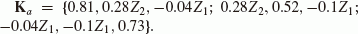Elastic tensor (in GPa) for dolomite rock is given byThe matrixdefines the anisotropic permeability of dolomite rock.

The matrix, defines the anisotropic elastic fluid-solid coupling matrix. This anisotropy is chosen with restriction that deviation from isotropy in any direction is not more than 20%. Similarly, the initial stress (in GPa) is assumed through the symmetric matrix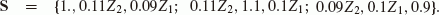This tensor restricts the initial stress up to maximum 1.1 GPa.

The above given sets of numerical values with Z1 = Z2 = 1 define the triclinic system of anisotropy for the medium and the initial stress. The values Z1 = 0, Z2 = 1 represent the monoclinic symmetry and the orthotropic symmetry is represented with Z1 = Z2 = 0.

## Rights and permissions

Reprints and Permissions

Sharma, M.D., Vashishth, A.K. Intrinsic attenuation from inhomogeneous waves in a dissipative anisotropic poroelastic medium. Earth Planet Sp 63, 89–101 (2011). https://doi.org/10.5047/eps.2010.12.004

• Revised:

• Accepted:

• Published:

• Issue Date:

### Key words

• Intrinsic attenuation
• inhomogeneous waves
• dissipative porous solid
• seismic anisotropy
• initial-stress
• anelasticity